# NPN: Help me understand how to choose Hfe, Ic, Vc "So That they can happen together"

#### babaliaris

Joined Nov 19, 2019
160
I'm trying for a year now to understand this and no matter how good I am at solving amplifier circuits at my University I still can't
figure this out. This is what I'm talking about:

In all my experiments I assume $$V_{BE} = 0.7V$$ because it seems to work and I do the calculations using
$$R_{1} = \frac{V_{cc}-V_{b}}{I_{b}}$$ , $$R_{2} = \frac{V_{cc}-V_{c}}{I_{c}}$$ , $$I_{b} = \frac{I_{c}}{B}$$

First try:
$$V_{c} = 0$$ , $$I_{c} = 100mA$$ , $$B = 10$$

$$R_{2} = \frac{10-0}{100mA} = 100 Ohms$$ , $$I_{b} = \frac{100mA}{10} = 10mA$$ , $$R_{1} = \frac{10-0.7}{10mA} = 930Ohms$$

And it works: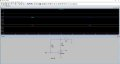Second try:
$$V_{c} = 0$$ , $$I_{c} = 100mA$$ , $$B = 800$$ (Max Hfe for BC547C)

$$R_{2} = \frac{10-0}{100mA} = 100 Ohms$$ , $$I_{b} = \frac{100mA}{800} = 0.125mA$$ , $$R_{1} = \frac{10-0.7}{0.125mA} = 74.4k$$

And it doesn't work. Ib is the same as my calculations but Ic and Vc that I requested are different.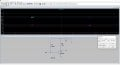Third try:
The previous probably didn't work because the following values can not exist at the same time when the transistor is operating:
$$V_{c} = 0$$ , $$I_{c} = 100mA$$ , $$B = 800$$ (Max Hfe for BC547C)

Now, what if I try to figure out the MAX Hfe that I can choose for $$I_{c} = 100mA$$?
$$V_{c} = 0$$ , $$I_{c} = 100mA$$ , $$B = ?$$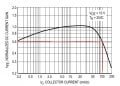The graph is telling me that for 100mAdc the max value of Hfe is 0.6 (normalized value) which means that any <= 0.6
should work with that current as long as there is a resistor in the collector. Of course if the transistor is operating by itself there is only
one value Hfe = 0.6 for a Ic = 100mA

$H_{fe} = B = H_{fe_{normalized}} \cdot [max - min] + min = 0.6 \cdot [800 - 420] + 420 = 648$

$$R_{2} = \frac{10-0}{100mA} = 100 Ohms$$ , $$I_{b} = \frac{100mA}{648} = 0.15mA$$ , $$R_{1} = \frac{10-0.7}{0.15mA} = 62k$$

And it doesn't work:
Again Ib is what I predicted with my math, but everything else is not!!!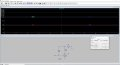The problem is definetly that these linear formulas:
$$R_{1} = \frac{V_{cc}-V_{b}}{I_{b}}$$ , $$R_{2} = \frac{V_{cc}-V_{c}}{I_{c}}$$ , $$I_{b} = \frac{I_{c}}{B}$$
mathematically speaking can take any range of values (except not zero in the denominator of course).

The reality though, is that the transistor is not linear and I must choose the values in such a way that they can "happen together".

How do I figure this out?
Thanks!

PS:
From my first try, you can see that I use Hfe = 10 and the LTspice is showing that this can happen since the circuit works as my math told me.
So what does the datasheet mean by these Hfe values: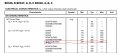Clearly from my experiments, I can have Hfe values less than 420.

#### Attachments

• 671 bytes Views: 3
Last edited:

#### ericgibbs

Joined Jan 29, 2010
18,226
hi baba,
Please post your LTS asc files so that we can compare results.
E

•babaliaris

#### DickCappels

Joined Aug 21, 2008
10,104
Just a note: the Hfe in the datasheets are not precise, they are only to give the designer an idea of what to expect. Especially with analog circuits, it is important to include some kind of feedback to stabilize the output. An emitter resistor provides negative feedback through the emitter, feedback from the collector signal to the base also works. Open loop circuits like this are for saturated switches.

•babaliaris

#### babaliaris

Joined Nov 19, 2019
160
From my experiments, it seems that when $$V_{C} = \frac{V_{CC}}{2}$$ then $$I_{C}$$ will approximately be half the max current while Hfe should also be half of its max value.

1) Now when Ic increases (towards max value) Vc goes to zero while Hfe goes to zero as well (saturation).
2) When Ic decreases Vc goes to Vcc and B goes towards its max value.

Is this true? This can explain why when I choose Ic=100mA, Vc=0, B=800 the transistor does not work as expected. When Ic goes high enough such as 100mA then yes, Vc has to go towards 0 to allow the max current but due to explanation 1) Hfe goes to zero as well so I can't choose 800 because it can't be this value.

If I could somehow see this in a graph or something and be able to see precisely what I can choose, that would be cool.

#### babaliaris

Joined Nov 19, 2019
160
By the way, does anyone know where I can learn about this stuff? An Udemy course or something else on the web? Or a book? I'm talking about a source that makes you a Master of transistors and circuit design, not only how to make the analysis on something that is already working.

It seems that Universities don't teach us everything.

How did you learn it?

#### Jony130

Joined Feb 17, 2009
5,475
First, notice that BC54xx family NPN translators have Ic_max= 100mA. So it is not wise to use such a "high current" (high for BC54xx transistor) that can easily damage the transistor.
Next, you must decide on which region you want to operate your BJT's.
If you want your BJT's to work as a saturated switch (Vce ≈ 0).

Then in a typical application any base current large than:

$$\Large I_B > \frac{\frac{V_{cc}}{R_c}}{\beta_{min} }$$ will saturate the BJT.

But for any real-world transistor, the β (Hfe) is not constant. Beta (Hfe) varies with Ic, Vce, temperature. And what is worse, every single transistor will have a different beta value.

Thus, to overcome this problem with beta and saturation we overdrive the base with a much large base current, we simply increase the base current well beyond:

$$\Large I_B > \frac{\frac{V_{cc}}{R_c}}{\beta_{min} }$$

We do this to make sure that we have enough base current to put the transistor well into saturation for every condition we have in our circuit.
Additional most BJT's vendors define saturation region when Ic/Ib = 10 (called Forced Beta). And the most datasheet show Vce_sat for Ic/Ib = 10
So, to be one hundred percent sure that your BJT will be in the saturation region you must use this so-called "forced beta" technique when choosing base resistor value.
Thus the typical forced beta value should be around 10...50. So there is nothing special about the "10" number.

But if you want Vce to be larger than 1V or more, the BJT's will work in active mode. But as you have already noticed the Hfe is not constant and hard to predict.
The only thing you can do is to take the geometric mean value of a Hfe and calculate the Rb and RC based on this assumption. Next, build the circuit and tweak the RB value on the testbench to get desired Vce value. This is why we don't use this type of "biasing circuit" in the real world. We use a voltage divider at the input and the emitter resistance.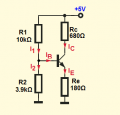•babaliaris

#### babaliaris

Joined Nov 19, 2019
160
Thank you for your answer. Now I would like for you to read the following statements and correct me if I'm wrong or answer yes if I'm correct:

Statements:
1) Hfe can not be predicted precisely but using the datasheets for specific Ic currents I can see a range that can be expected.

2) If I choose Ic and Vc and try to calculate Rc, if Ic, Vc were not chosen so that "they can happen together" I will get nonsense results. For example Ic=maxI, Vc = Vcc/2, this CAN NOT happen since Vc must be zero to saturate the NPN and to be able to get the max current.

3) So from the 2) conclusion -> Choosing the operation of the transistor: I should choose a Vc and a known Rc in order to calculate the correct Ic. I know that Rc affects the gain, but let's say we don't care about it for now.

Questions:
A) After Choosing Vc, Rc, and having calculated Ic, what am I going to do about Ib? Since I can't use a value for Hfe I can't find Ib which means I can't calculate Rb.

B) You are talking about Vce and not Vc. If I have a resistor Re in the emitter which means that Vc is not equal to Vce anymore when choosing the operation of the transistor, should I choose Vce or Vc? In class, our professor told us that we use Vc. For example, if Vc=Vcc/2 we are in the active region.

The only thing you can do is to take the geometric mean value of a Hfe and calculate the Rb and RC based on this assumption. Next, build the circuit and tweak the RB value on the testbench to get desired Vce value. This is why we don't use this type of "biasing circuit" in the real world. We use a voltage divider at the input and the emitter resistance.
How do I figure out the geometric mean value of a Hfe? I know the formula for the geometric mean, but which values do I choose from the datasheet? I'm attaching the datasheet if you are kind enough to show me the values that I need to calculate the mean.

#### Attachments

• 195.9 KB Views: 2
Last edited:

#### Jony130

Joined Feb 17, 2009
5,475
1) Hfe can not be predicted precisely but using the datasheets for specific Ic currents I can see a range that can be expected.
Yes.

If I choose Ic and Vc and try to calculate Rc, if Ic, Vc were not chosen so that "they can happen together" I will get nonsense results.
No, surely you can pick any Vc you want, the same is true for Ic.
For example, I can pick Ic = Vcc/2 and Ic =1mA. or 10mA or 50mA. But next, I have to check if the transistor will survive the choice I made. Vc must be lower than Vce max, and the same is true for the collector current. Ic lower than Ic_max (given in datasheet for a given BJT). And power dissipation also needs to be checked (P = Vce * Ic).

For example Ic=maxI, Vc = Vcc/2, this CAN NOT happen since Vc must be zero to saturate the NPN and to be able to get the max current.
How do you define "max current"? The datasheet value? Or in aplication Ic_max = Vcc/Rc?

3) So from the 2) conclusion -> Choosing the operation of the transistor: I should choose a Vc and a known Rc in order to calculate the correct Ic. I know that Rc affects the gain, but let's say we don't care about it for now.
This will vary, it is an application-dependent situation. Sometimes we choose Ic and Vc and based on this assumption we calculate Rc value.

Try to read this example and post #11 as well.

A) After Choosing Vc, Rc, and having calculated Ic, what am I going to do about Ib? Since I can't use a value for Hfe I can't find Ib which means I can't calculate Rb.
If you plan to work in the active region then you are forced to use a voltage divider + Re resistor. And in this case, you pick Hfe_min for calculation purposes.

B) You are talking about Vce and not Vc. If I have a resistor Re in the emitter which means that Vc is not equal to Vce anymore when choosing the operation of the transistor, should I choose Vce or Vc? In class, our professor told us that we use Vc. For example, if Vc=Vcc/2 we are in the active region.
Well, in this case, Vce = Vc - Ve. And to get the maximum swing we simply do this Vc = (Vcc - Ve)/2

How do I figure out the geometric mean value of a Hfe?
For low current, the typical gain is 270 and 520 for a "medium" current. Thus Hfe = √(270*520) ≈ 370

•babaliaris

#### babaliaris

Joined Nov 19, 2019
160
For low current, the typical gain is 270 and 520 for a "medium" current. Thus Hfe = √(270*520) ≈ 370
And in this case, you pick Hfe_min for calculation purposes.
Can you show me how to find these values in the datasheet? I'm talking about 270, 520, and Hfe_min.
So I can know how to find them out, in case I'm using a different transistor.

#### Attachments

• 195.9 KB Views: 3

#### crutschow

Joined Mar 14, 2008
33,350
It still seems like you are still trying to use an analysis approach to synthesizing a circuit.
You generally want to use minimum gain values for the design, while designing it be relative insensitive to the maximum gain the transistor may have.
Good design uses worst-case values for the device parameters, so it will work with those as well as nominal or maximum parameters.
How did you learn it?
I also had no experience in designing circuits, as most of what I was taught was analysis, as your training has been.
I mostly learned by just doing some designs, which forced me to determine and generate the requirements for the design.
You can't really do a design unless you establish what the design has to do.
Saying you want to design an amplifier isn't sufficient.
You need to determine what all the amplifier requirements are.
Sometimes you will find requirements that are incompatible, or difficult to reasonably achieve, so you go back and redo them.
I think you've already encountered some of that in your design attempt.
And developing an intuitive understand how circuits work (by looking at designs already built) is very helpful in doing a new design.

•babaliaris

#### babaliaris

Joined Nov 19, 2019
160
Thank you, guys! I think I'm starting to realize what is going on.

About the negative feedback bias, I tried to think of a theory of how it works to keep Hfe constant, thus making my amplifier independent of Hfe, and I would like you to tell me if I'm correct or close to the truth:

$$H_{fe} = \frac{I_{c}}{I_{b}}$$ , $$I_{C} = H_{fe} \cdot I_{B}$$ , $$I_{E} = \frac{(H_{fe} +1)}{H_{fe}} \cdot I_{C}$$

If Ic and Ie (which are proportional) somehow get increased, then the voltage drop on Re increases which means Ve increases which means $$V_{BE} = V_{B} - V_{E}$$ decreases. But in order for the transistor to keep operating, Vbe must somehow be increased back to 0.7, so the Ib must increase as well in order for this to happen.

So if for some magical reason the increase of Ic, Ie results in a same increase in Ib then the fraction $$H_{fe} = \frac{I_{c}}{I_{b}}$$ stays the same.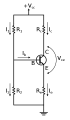#### babaliaris

Joined Nov 19, 2019
160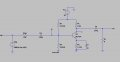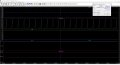Wtf is going on? I'm getting so much smaller Vo than Vsig, this does the opposite of amplifying the signal.

I designed it assuming the following Values (I found them in an Excersise from my University):
Vcc = 10v
Ic = 2mA
Vbe = 0.7
Iri = Ir2 = 0.2mA
vb = Vcc / 3
hfe = 220
Rsig = 10k
RL = 8k

Then I calculated Rc, Re, R1, R2.

In the bias calculations, only Ib was wrong. I found ib = 9μΑ while in the simulation Ib_real = 4.3μA
Also, Hfe is different in the simulation (about 464) which makes sense since the datasheet says in active mode for Ic=2mA Hfe can be [420, 800].

I calculated the capacitance of each capacitor (except Ce because I thought it doesn't matter since there is no resistor in series, so I used a random value) using c$$C = \frac{1}{2 \pi f R}$$ which I saw from a post that @Jony130 made which doesn't make sense to me because $Z = R + j \frac{1}{2 \pi f c}$ can't be solved for C since it's a complex number.

What are the spikes in the Gain plot? What causes the amp to not work?

I suspect the problem is in the random value of Ce that probably blocks the 15k frequency. If yes, how can I choose the capacitance of Ce?

Thanks.

PS: From the purple plot (Hfe), I believe, I can see how the negative feedback (from Re) tries to stabilize Hfe.

#### Attachments

• 3.8 KB Views: 1
Last edited:

#### babaliaris

Joined Nov 19, 2019
160
I believe it is: $$Z_{sig} = 10k - j \frac{1}{2 \pi \cdot 15k \cdot 1.06n} <=> Z_{sig} = (10 -j10.063 )kΩ$$

#### babaliaris

Joined Nov 19, 2019
160

#### ericgibbs

Joined Jan 29, 2010
18,226
hi baba,
You are overthinking the problem.Zc=10014.82 ohms.

You should now be able to realize why your amplifier has no gain at 15kHz.

The AC plot shows a -50dB loss at 15kHz.

E

•babaliaris

#### ericgibbs

Joined Jan 29, 2010
18,226
hi baba,
It is always important that you specify the required Gain and Bandwidth of your amplifier design.

What is your target design specification.?
E

•babaliaris

#### babaliaris

Joined Nov 19, 2019
160
So you are telling me that the Impedance of Vsig is about 10k which is what I chose for Rsig in order to model the internal resistance of the signal source.

By doing the math I figure out why this is happening: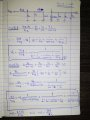The most important thing is that Rsig is in the denominator of the first fraction. So a big rise in Rsig results in a lower Gain.

I believe this is the reason. I will try now in the simulation to reduce Rsig and see what will happen.

#### ericgibbs

Joined Jan 29, 2010
18,226

#### Attachments

• 1.6 KB Views: 1